# Analysis of Algorithm

## Analysis of Algorithm

In this article, I am going to discuss the Analysis of Algorithm in Data Structure as well as why it is important to Analysis the Algorithm. Please read our previous article where we gave a brief introduction to the Algorithm. At the end of this article, you will understand the following pointers in detail.

1. Why Analysis of Algorithm?
2. The goal of Analysis of Algorithms.
3. What is Running Time Analysis?
4. How to compare Algorithms?
5. Does Asymptotic Analysis always work?
6. What is the Rate of Growth?
7. Types of Analysis
##### Why Analysis of Algorithm?

Algorithm analysis helps to determine the best among others in terms of time and space consumed. For example:- Going from one place to another, there is various way to travel. Like Bus, Train, Flight, Car, etc.

But we will select the best mode which is cost-efficient and time-consuming, depends on the situation. Similarly, In computer science to sort an array there are various ways or algorithms like insertion sort, selection sort, quick sort, merge sort, etc.

##### The goal of Analysis of Algorithms

The Goal of the analysis of algorithms is to compare algorithms (for solutions) mainly in terms of running time but also in terms of other factors (e.g. memory, developer’s effect, etc.)

##### What is Running Time Analysis?

It is the processing time vs the size of the input. The input may be of different types based on problems. Commons inputs are

1. Size of array
2. Number of elements in a matrix
3. Polynomial degree
4. Numbers of bits in binary representation
5. Edges and Vertices in a graph
##### How to compare Algorithms?

One native way of doing this is – implement both the algorithms and run the two programs on your computer for different inputs and see which one takes less time. There are many problems with this approach to the analysis of algorithms.

1. It might be possible that for some inputs, the first algorithm performs better than the second. And for some inputs second performs better.
2. It might also be possible that for some inputs, the first algorithm performs better on one machine and the second works better on other machines for some other inputs.

Asymptotic Analysis is a big idea that handles the above issues in analyzing algorithms. In Asymptotic Analysis, we evaluate the performance of an algorithm in terms of input size (we don’t measure the actual running time). We calculate, how the time (or space) taken by an algorithm increases with the input size.

This kind of comparison is independent of machine time, programming type, etc.

##### Example to understand this concept:

For example, let us consider the search problem (searching a given item) in a sorted array. One way to search is Linear Search (order of growth is linear) and the other way is Binary Search (order of growth is logarithmic).

To understand how Asymptotic Analysis solves the above-mentioned problems in analyzing algorithms, let us say we run the Linear Search on a fast computer A and Binary Search on a slow computer B and we pick the constant values for the two computers so that it tells us exactly how long it takes for the given machine to perform the search in seconds.

Let’s say the constant for A is 0.2 and the constant for B is 1000 which means that A is 5000 times more powerful than B. For small values of input array size n, the fast computer may take less time. But, after a certain value of input array size, the Binary Search will definitely start taking less time compared to the Linear Search even though the Binary Search is being run on a slow machine.

The reason is the order of growth of Binary Search with respect to input size is logarithmic while the order of growth of Linear Search is linear. So the machine-dependent constants can always be ignored after a certain value of input size.

Here are some running times for this example:
Linear Search running time in seconds on A: 0.2 * n
Binary Search running time in seconds on B: 1000*log(n)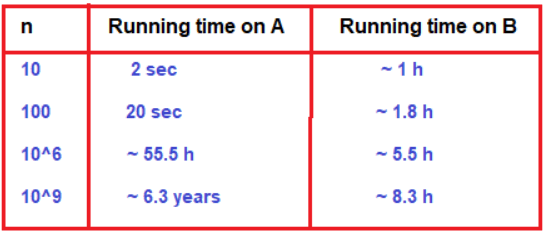##### Does Asymptotic Analysis always work?

Asymptotic Analysis is not perfect, but that’s the best way available for analyzing algorithms. For example, say there are two sorting algorithms that take 1000nLogn and 2nLogn time respectively on a machine. Both of these algorithms are asymptotically the same (order of growth is nLogn). So, With Asymptotic Analysis, we can’t judge which one is better as we ignore constants in Asymptotic Analysis.

Also, in Asymptotic analysis, we always talk about input sizes larger than a constant value. It might be possible that those large inputs are never given to your software and an algorithm that is asymptotically slower, always performs better for your particular situation. So, you may end up choosing an algorithm that is Asymptotically slower but faster for your software.

##### What is the Rate of Growth?

The rate at which running time increases as a function of input is called the rate of growth.

We know that for the growth of a function, the highest order term matters the most e.g., the term c1n2 in the function c1n2+c2n+c3 and thus we can neglect the other terms and even the coefficient of the highest order term i.e., c1 (assuming coefficients are neither too large nor too small).

Even with these approximations, we will be able to know about the rate of the growth of our function and this is enough information to keep in our mind while developing an algorithm.

##### Commonly used rate of Growths in decreasing order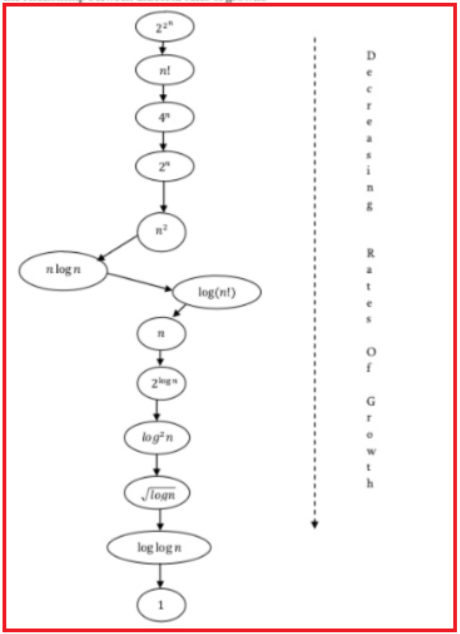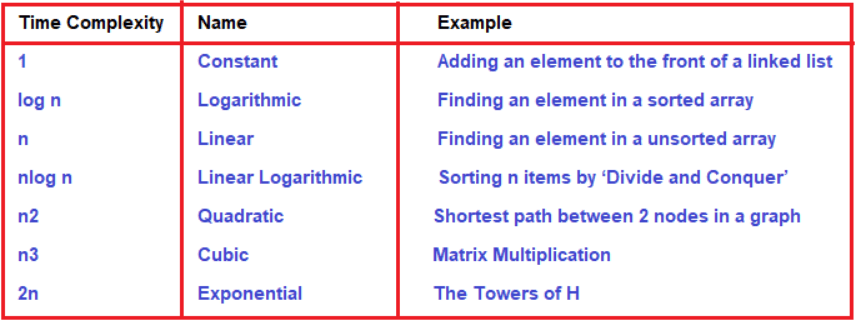##### Commonly used functions and Their comparison: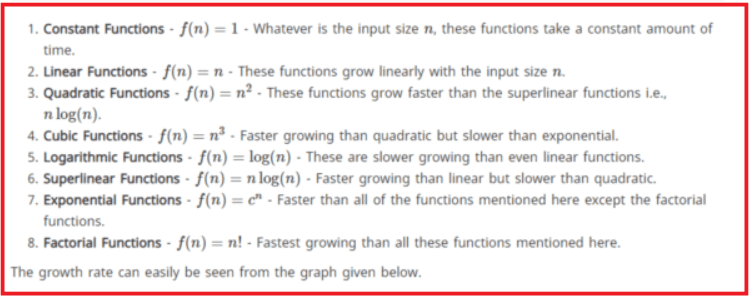and the problem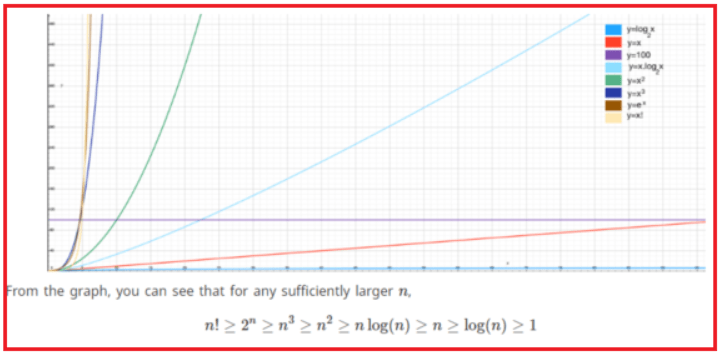##### Types of Analysis

To analyze the given algorithm we need to know on what inputs the algorithm is taking less time (performing well) and on what inputs the algorithm is taking a huge time. We know that an algorithm can be represented in the form of expression. That means we represent the algorithm with multiple expressions: one for the case where it is taking less time and others for the case where it is taking more time. In general-

1. Worst Case:- Defines the input for which the algorithm takes a huge time. Input is the one for which the algorithm runs slower.
2. Best Case:- Defines the input for which algorithm takes the lowest time. Input is the one for which algorithm works fastest.
3. Average Case:- Provides a prediction about the running time of the algorithm. Assumes that the input is random.

Lower Bound <= Average Time <= Upper Bound
For a given algorithm, we can represent best, worst, and average cases in the form of expression.

Example:-
f(n) = n2 + 500, for worst-case
f(n) = n + 100n + 500, for best case

Similarly, we can define the average case too.